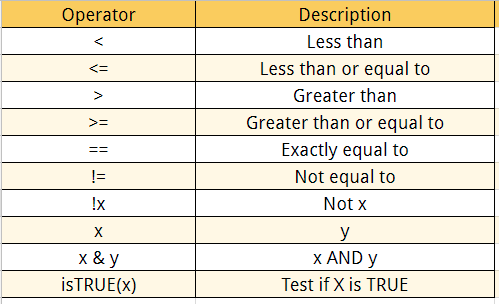# R Logical Operators

Q:
R Logical Operators

## ▼ Show 1 Answer

0 votes

With logical operators, we want to return values inside the vector based on logical conditions. Following is a detailed list of logical operators available in RThe logical statements in R are wrapped inside the []. We can add many conditional statements as we like but we need to include them in a parenthesis. We can follow this structure to create a conditional statement:

`variable_name[(conditional_statement)]`

With variable_name referring to the variable, we want to use for the statement. We create the logical statement i.e. variable_name > 0. Finally, we use the square bracket to finalize the logical statement. Below, an example of a logical statement.

Example 1:

```# Create a vector from 1 to 10
logical_vector <- c(1:10)
logical_vector>5```

Output:

`## FALSE FALSE FALSE FALSE FALSE  TRUE  TRUE  TRUE  TRUE  TRUE`

In the output above, R reads each value and compares it to the statement logical_vector>5. If the value is strictly superior to five, then the condition is TRUE, otherwise FALSE. R returns a vector of TRUE and FALSE.

Learn More with Madanswer

0 votes
+2 votes
+2 votes
0 votes
0 votes
0 votes
0 votes